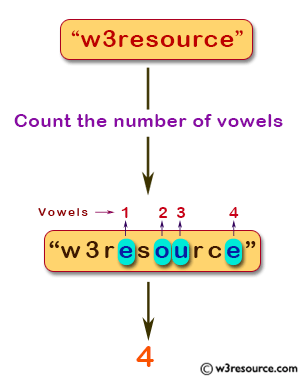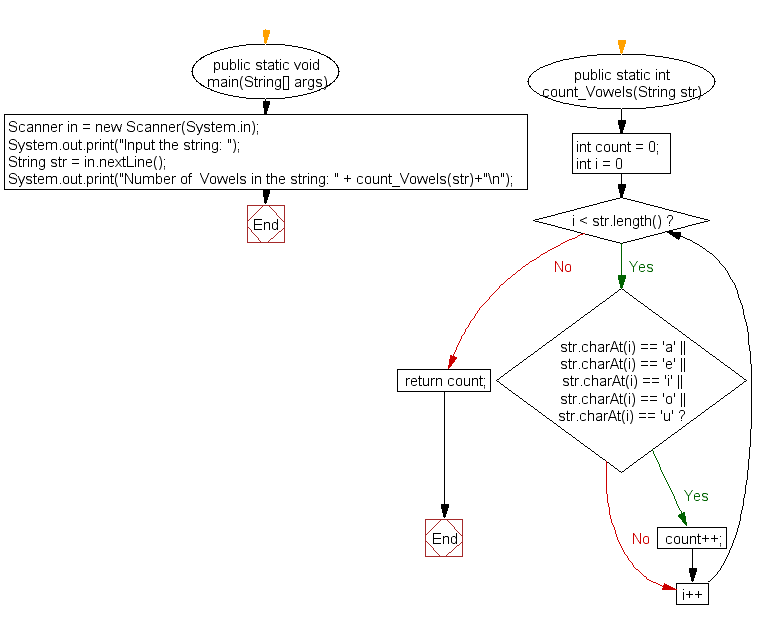﻿ Java exercises: Count all vowels in a string - w3resource# Java Method Exercises: Count all vowels in a string

## Java Method: Exercise-4 with Solution

Write a Java method to count all vowels in a string.

Sample Solution:

Test Data:
Input the string: w3resource

Pictorial Presentation:Sample Solution:

Java Code:

``````import java.util.Scanner;
public class Exercise4 {
public static void main(String[] args)
{
Scanner in = new Scanner(System.in);
System.out.print("Input the string: ");
String str = in.nextLine();

System.out.print("Number of  Vowels in the string: " + count_Vowels(str)+"\n");
}
public static int count_Vowels(String str)
{
int count = 0;
for (int i = 0; i < str.length(); i++)
{
if (str.charAt(i) == 'a' || str.charAt(i) == 'e' || str.charAt(i) == 'i'
|| str.charAt(i) == 'o' || str.charAt(i) == 'u')
{
count++;
}
}
return count;
}
}
```
```

Sample Output:

```Input the string: w3resource
Number of  Vowels in the string: 4
```

Flowchart:Java Code Editor: